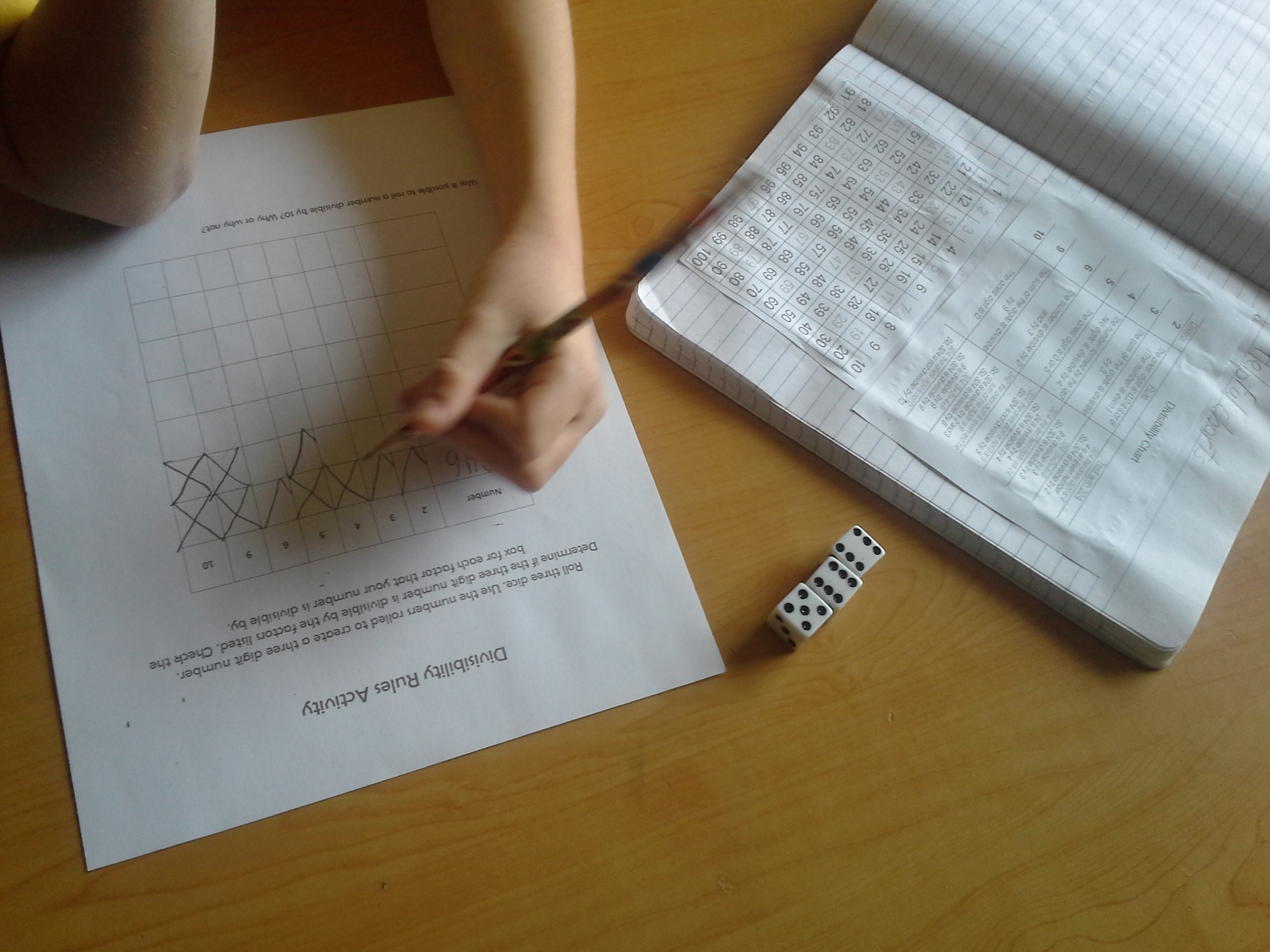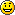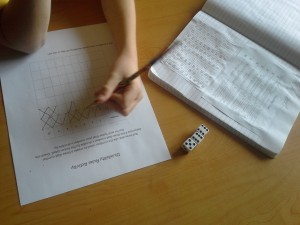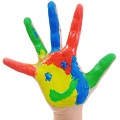# Divisibility Rules Activity

As your child progresses in math, divisibility rules come in very handy. This activity focuses on the divisibility rules for 2, 3, 4, 5, 6, 9, and 10. There are rules for more numbers, such as 8, 9, and 11 etc., however, I will focus on the ones previously listed. With these first seven rules learned your child will have a strong start with the most commonly used, and taught, divisibility rules. There are many uses for divisibility rules, such as prime factorization, fraction work, and of course division.

First, print out a divisibility rules chart for your child to use a reference. I created a very simple chart that is small enough to cut out and paste in a math notebook if needed. Show your child how s/he can determine if number is divisible by either 2, 3, 4, 5, 6, 9, or 10 by following the rule for each one listed. I would go through them one at a time, making sure your child understands as you go along.

Materials needed:

3 Dice

Pencil

Activity:

1. Roll 3 dice.
2. Put the dice together in a line. Use the numbers on the dice to form a three digit number.
3. Referencing the divisibility rules chart, determine if the number is divisible by 2, 3, 4, 5, 6, 9, and 10.  Your child may need your help for the first one to get the hang of it.
4. Put an X in the box of any divisible factors. For example, 246 is divisible by 2, 3, and 6 so the child should check the boxes for 2, 3, and 6.
5. Repeat the process with more three digit numbers.

By the end of the activity, your child should become more and more comfortable with the rules and may even have them memorized.

Here’s a picture of my son doing the activity.  As you can see he modified the instructions by using check marks and XsTry this activity next: